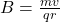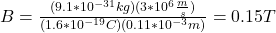## 3) An electron moves in a circular orbit of radius 0.11 mm, counterclockwise as you look down at it, moving in a plane above and parallel to

Question

3) An electron moves in a circular orbit of radius 0.11 mm, counterclockwise as you look down at it, moving in a plane above and parallel to the plane of your exam paper. The velocity of the electron is v = 3 × 106m/s. a) Find the magnetic field due to the electron at the center of the circle. b) Is this magnetic field going into the page at the center of the circle or coming towards your face at the center of the circle as you look down at the electron?

in progress 0
2 months 2021-07-15T08:08:43+00:00 1 Answers 4 views 0

B = 0.15 T

Explanation:

To find the magnitude of the magnetic field you use the following formula:( 1 )

m: mass of the electron = 9.1*10^-31 kg

v: velocity of the electron = 3*10^6 m/s

q: charge = 1.6*10^-19

r: radius = 0.11mm = 0.11*10^-3 m

You replace the values of the parameters in the equation (1).the magnetic field has a magnitude of 0.15 T